# 如何基于梯度优化分析冰川流动？

2018年 7月 17日

### 使用 COMSOL Multiphysics® 计算梯度

\frac{\partial j}{\partial \alpha_k}=\frac{\partial j}{\partial u}\cdot\frac{\partial u}{\partial \alpha_k}.

\frac{\partial M}{\partial u}\cdot\frac{\partial u}{\partial \alpha_k}=\frac{\partial M}{\partial \alpha_k}.

\frac{\partial j}{\partial \alpha_k}=\frac{\partial j}{\partial u}\cdot\left(\frac{\partial M}{\partial u}\right)^{-1}\cdot\frac{\partial M}{\partial \alpha_k}.

u^*=\frac{\partial j}{\partial u}\cdot\left(\frac{\partial M}{\partial u}\right)^{-1}

\frac{\partial M}{\partial u}^T u^*=\frac{\partial j}{\partial u}.

### 基于梯度优化的冰川分析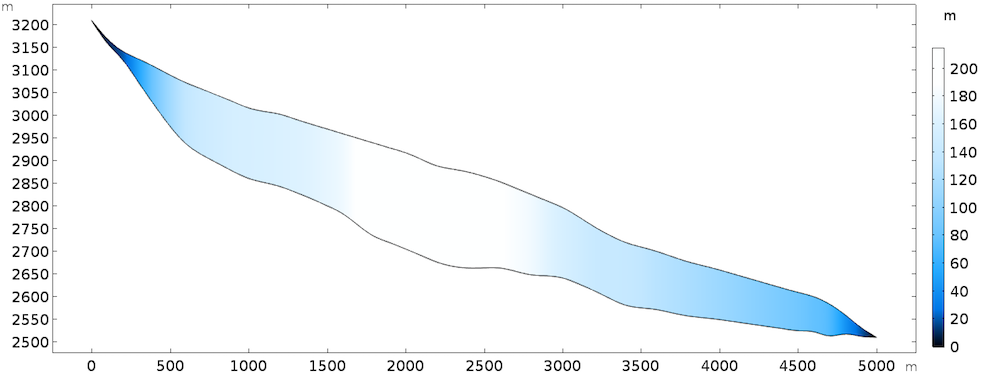L_s=
\begin{cases}
20m \mbox{ if } 0\leq x \leq 1000 \\
50m \mbox{ if } 1000\leq x \leq 4000 \\
200m \mbox{ if } 4000\leq x \leq 5000
\end{cases}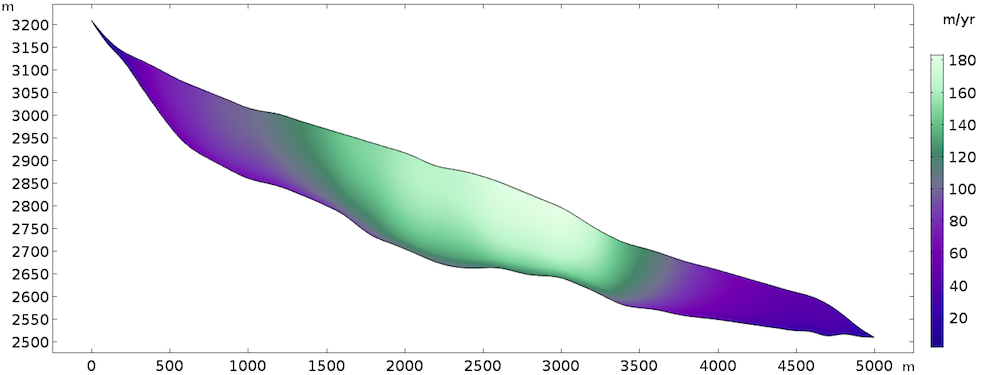### 执行灵敏度分析

j=\int_{\Gamma_s} (u_t-u_{obs})^2 ds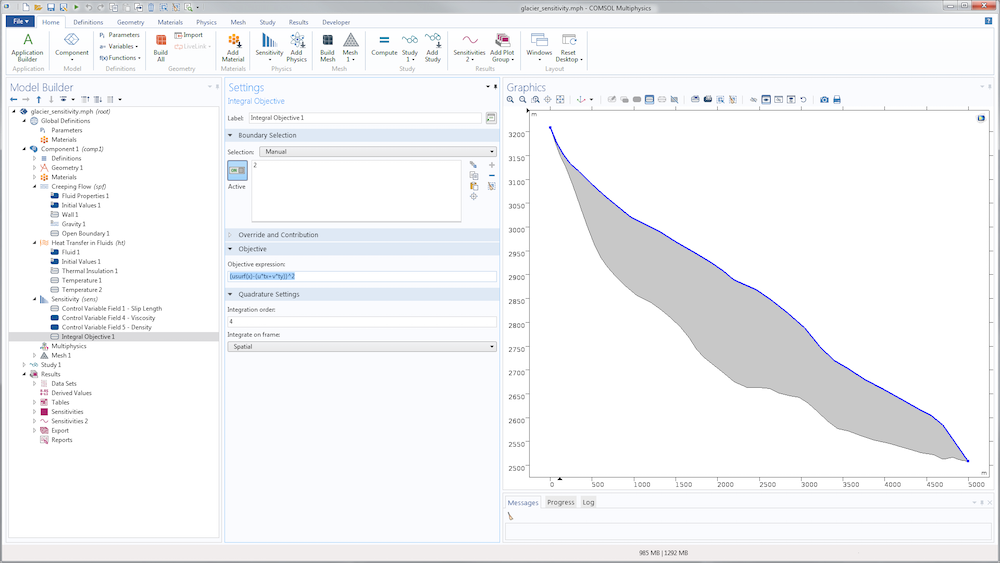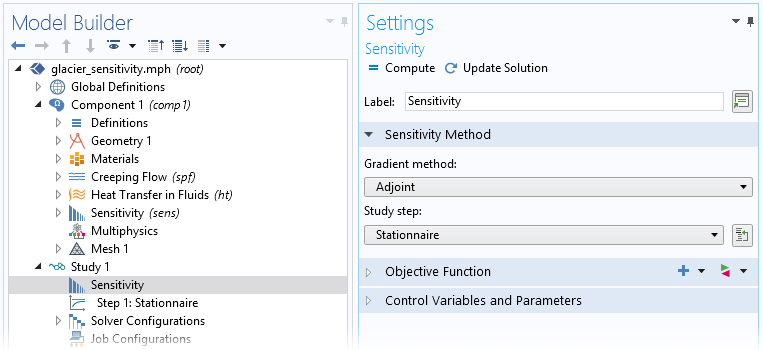\widetilde{\alpha_k}^*=\left|\frac{\widetilde{\alpha_k}}{h_k}\frac{\alpha_0} {j(\alpha_0}\right|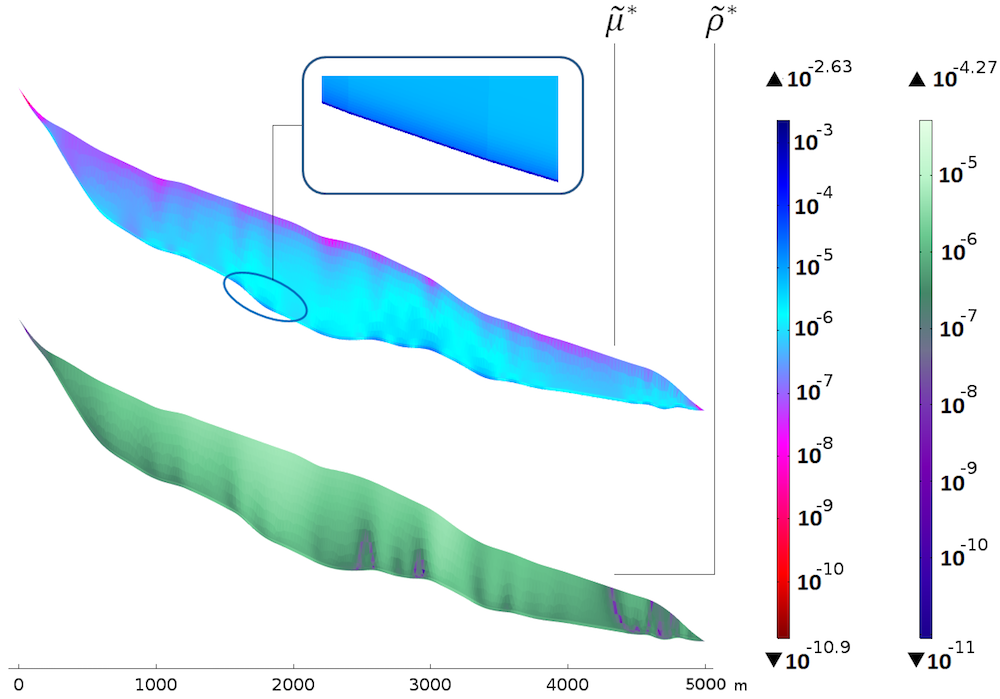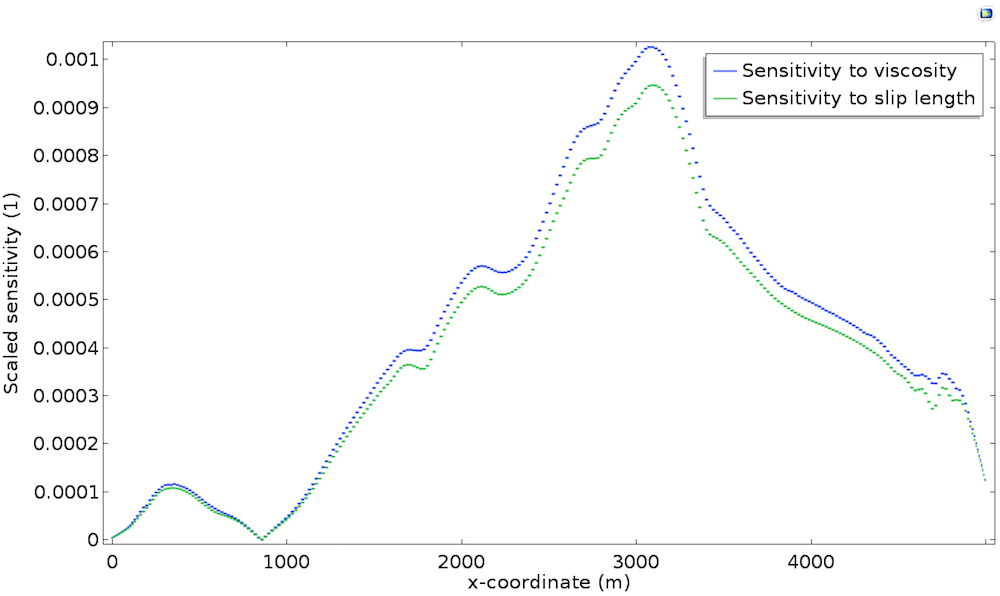1. 出现在滑移长度和黏度上，并仅位于基岩上
2. 与基岩的地形有很强的相关性(使它们既重要又难以观测)

### 数据同化

1. 沿基岩的滑移长度
2. 基岩的形状d_b=d_c\frac{y-y_s} {y_b-y_s}